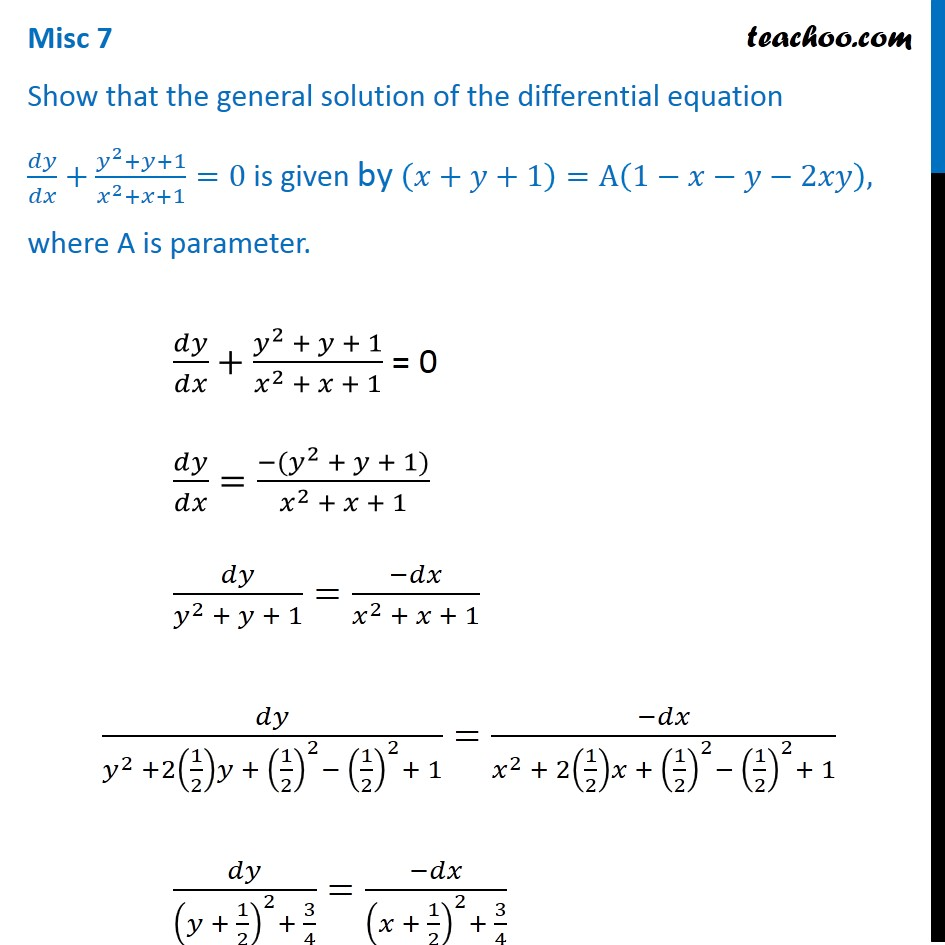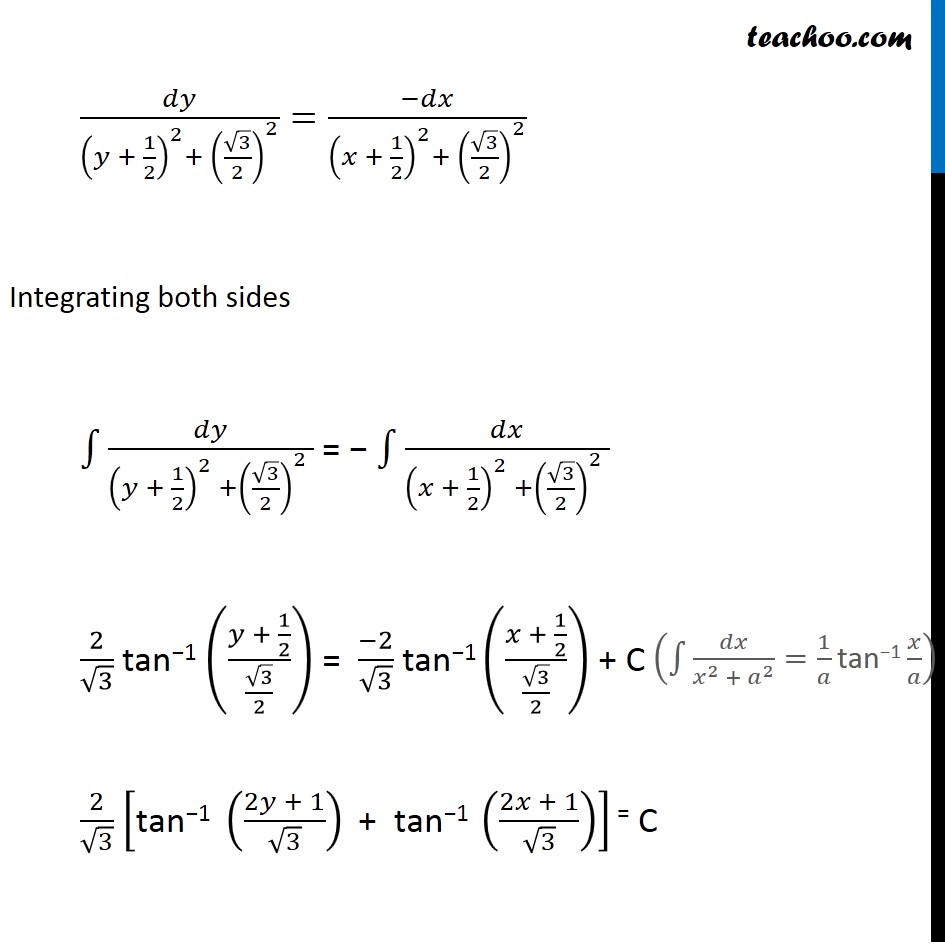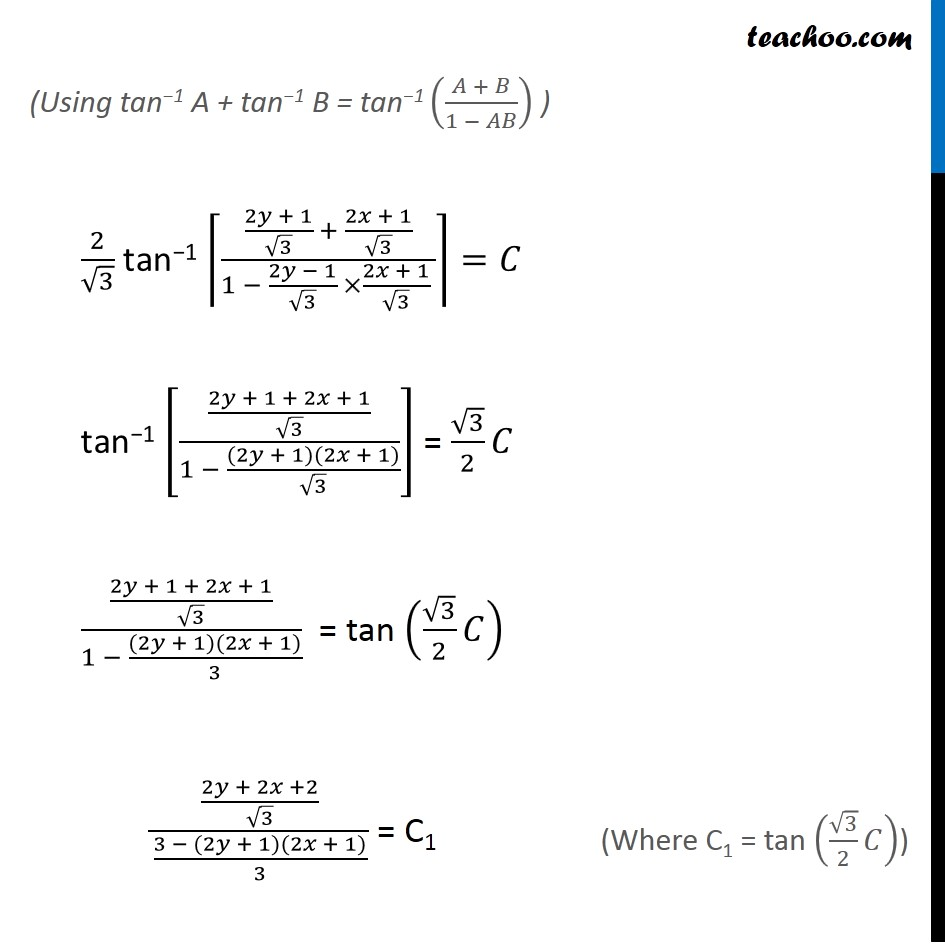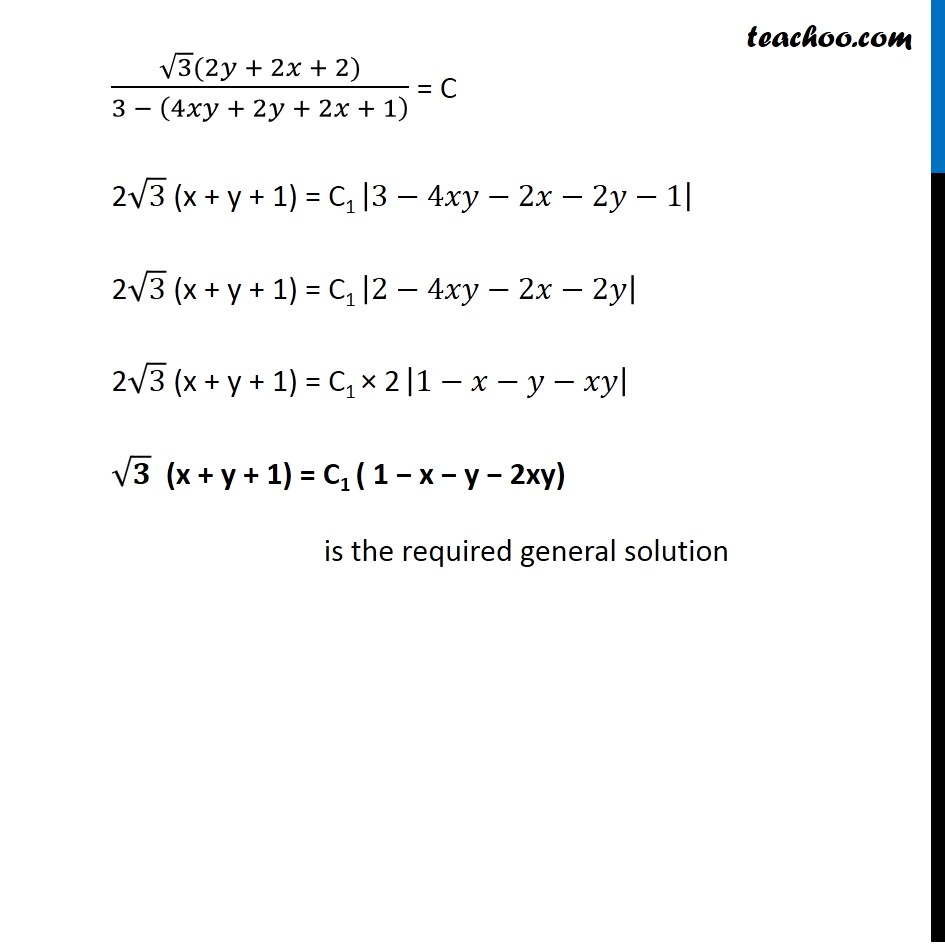Miscellaneous

Chapter 9 Class 12 Differential Equations
Serial order wiseLearn in your speed, with individual attention - Teachoo Maths 1-on-1 Class

### Transcript

Misc 5 Show that the general solution of the differential equation 𝑑𝑦/𝑑𝑥+(𝑦^2+𝑦+1)/(𝑥^2+𝑥+1)=0 is given by (𝑥+𝑦+1)=A(1−𝑥−𝑦−2𝑥𝑦), where A is parameter. 𝑑𝑦/𝑑𝑥+(𝑦^2 + 𝑦 + 1)/(𝑥^2 + 𝑥 + 1) = 0 𝑑𝑦/𝑑𝑥=(−(𝑦^2 + 𝑦 + 1))/(𝑥^2 + 𝑥 + 1) 𝑑𝑦/(𝑦^2 + 𝑦 + 1)=(−𝑑𝑥)/(𝑥^2 + 𝑥 + 1) 𝑑𝑦/(𝑦^2 +2(1/2)𝑦 + (1/2)^2− (1/2)^2+ 1)=(−𝑑𝑥)/(𝑥^2 + 2(1/2)𝑥 + (1/2)^2− (1/2)^2+ 1) 𝑑𝑦/((𝑦 + 1/2)^2+ 3/4)=(−𝑑𝑥)/((𝑥 + 1/2)^2+ 3/4) 𝑑𝑦/((𝑦 + 1/2)^2+ (√3/2)^2 )=(−𝑑𝑥)/((𝑥 + 1/2)^2+ (√3/2)^2 ) Integrating both sides ∫1▒𝑑𝑦/((𝑦 + 1/2)^2 +(√3/2)^2 ) = − ∫1▒𝑑𝑥/((𝑥 + 1/2)^2 +(√3/2)^2 ) 2/√3 tan−1 ((𝑦 + 1/2)/(√3/2)) = (−2)/√3 tan−1 ((𝑥 + 1/2)/(√3/2)) + C 2/√3 ["tan−1 " ((2𝑦 + 1)/√3)" + tan−1 " ((2𝑥 + 1)/√3)] = C (∫1▒𝑑𝑥/(𝑥^2 + 𝑎^2 )=1/𝑎 "tan−1" 𝑥/𝑎) (Using tan−1 A + tan−1 B = tan−1 ((𝐴 + 𝐵)/(1 − 𝐴𝐵)) ) 2/√3 "tan−1" ⌈((2𝑦 + 1)/√3 + (2𝑥 + 1)/√3)/(1 − (2𝑦 − 1)/√3 ×(2𝑥 + 1)/√3 )⌉=𝐶 "tan−1" [((2𝑦 + 1 + 2𝑥 + 1)/√3)/(1 − ((2𝑦 + 1)(2𝑥 + 1))/√3)] = √3/2 𝐶 ((2𝑦 + 1 + 2𝑥 + 1)/√3)/(1 − ((2𝑦 + 1)(2𝑥 + 1))/3) = tan (√3/2 𝐶) ((2𝑦 + 2𝑥 +2)/√3)/((3 − (2𝑦 + 1)(2𝑥 + 1))/3) = C1 (Where C1 = tan (√3/2 𝐶)) (√3(2𝑦 + 2𝑥 + 2))/(3 − (4𝑥𝑦 + 2𝑦 + 2𝑥 + 1) ) = C 2√3 (x + y + 1) = C1 |3−4𝑥𝑦−2𝑥−2𝑦−1| 2√3 (x + y + 1) = C1 |2−4𝑥𝑦−2𝑥−2𝑦| 2√3 (x + y + 1) = C1 × 2 |1−𝑥−𝑦−𝑥𝑦| √𝟑 (x + y + 1) = C1 ( 1 − x − y − 2xy) is the required general solution